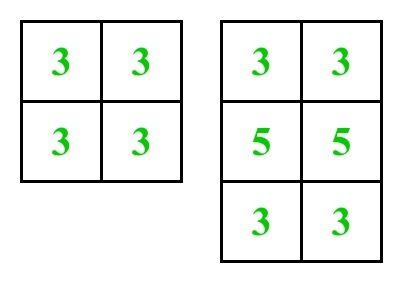# Sum of the count of number of adjacent squares in an M X N grid

Given an M × N matrix. The task is to count the number of adjacent cells and calculate their sum.
Two cells are said to be connected if they are adjacent to each other horizontally, vertically, or diagonally.
Examples :

Input : m = 2, n = 2
Output : 12
Input : m = 3, n = 2
Output: 22
See the below diagram where numbers written on it denotes number of adjacent squares.Approach:
In a m X n grid there can be 3 cases:

• Corner cells touch 3 cells, and there are always 4 corner cells.
• Edge cells touch 5 cells, and there are always 2 * (m+n-4) edge cells.
• Interior cells touch 8 cells, and there are always (m-2) * (n-2) interior cells.

Therefore,

```Sum = 3*4 + 5*2*(m+n-4) + 8*(m-2)*(n-2)
= 8mn - 6m - 6n +4```

Below is the implementation of the above approach:

## C++

 `// C++ implementation of the above approach`   `#include ` `using` `namespace` `std;`   `// function to calculate the sum of all cells adjacent value` `int` `sum(``int` `m, ``int` `n)` `{` `    ``return` `8 * m * n - 6 * m - 6 * n + 4;` `}`   `// Driver program to test above` `int` `main()` `{` `    ``int` `m = 3, n = 2;` `    ``cout << sum(m, n);`   `    ``return` `0;` `}`

## Java

 `// Java implementation of the above approach ` `class` `GFG ` `{` `    `  `    ``// function to calculate the sum ` `    ``// of all cells adjacent value ` `    ``static` `int` `sum(``int` `m, ``int` `n) ` `    ``{ ` `        ``return` `8` `* m * n - ``6` `* m - ``6` `* n + ``4``; ` `    ``} ` `    `  `    ``// Driver Code` `    ``public` `static` `void` `main (String[] args)` `    ``{ ` `        ``int` `m = ``3``, n = ``2``; ` `        ``System.out.println(sum(m, n)); ` `    ``} ` `}`   `// This Code is contributed by AnkitRai01`

## Python3

 `# Python3 implementation of the above approach`   `# function to calculate the sum` `# of all cells adjacent value` `def` `summ(m, n):` `    ``return` `8` `*` `m ``*` `n ``-` `6` `*` `m ``-` `6` `*` `n ``+` `4`   `# Driver Code` `m ``=` `3` `n ``=` `2` `print``(summ(m, n))`   `# This code is contributed by Mohit Kumar`

## C#

 `// C# implementation of the above approach ` `using` `System;` `class` `GFG ` `{` `    `  `    ``// function to calculate the sum ` `    ``// of all cells adjacent value ` `    ``static` `int` `sum(``int` `m, ``int` `n) ` `    ``{ ` `        ``return` `8 * m * n - 6 * m - 6 * n + 4; ` `    ``} ` `    `  `    ``// Driver Code` `    ``public` `static` `void` `Main (String []args)` `    ``{ ` `        ``int` `m = 3, n = 2; ` `        ``Console.WriteLine(sum(m, n)); ` `    ``} ` `}`   `// This code is contributed by andrew1234`

## Javascript

 ``

Output:

`22`

Time Complexity:Auxiliary Space: O(1)

Feeling lost in the world of random DSA topics, wasting time without progress? It's time for a change! Join our DSA course, where we'll guide you on an exciting journey to master DSA efficiently and on schedule.
Ready to dive in? Explore our Free Demo Content and join our DSA course, trusted by over 100,000 geeks!

Previous
Next